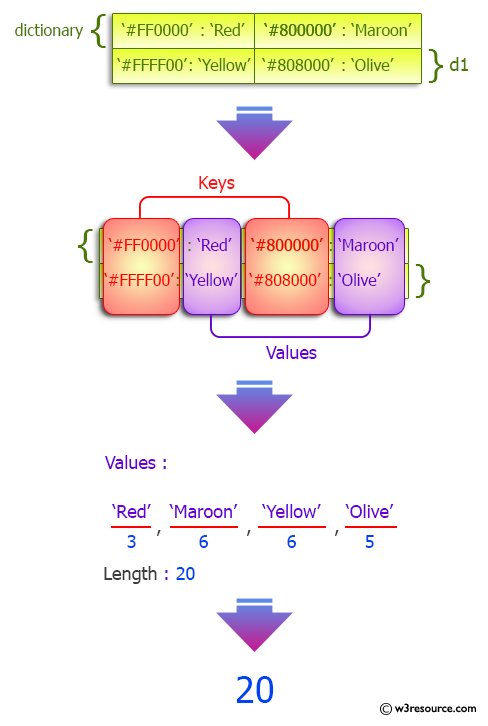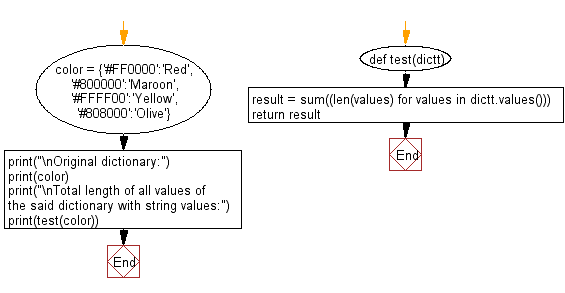﻿ Python: Total length of all values of a given dictionary with string values - w3resource# Python: Total length of all values of a given dictionary with string values

## Python dictionary: Exercise-65 with Solution

Write a Python program to get the total length of all values of a given dictionary with string values.

Sample Solution:

Python Code:

``````def test(dictt):
result = sum((len(values) for values in dictt.values()))
return result
color = {'#FF0000':'Red', '#800000':'Maroon', '#FFFF00':'Yellow', '#808000':'Olive'}
print("\nOriginal dictionary:")
print(color)
print("\nTotal length of all values of the said dictionary with string values:")
print(test(color))
```
```

Sample Output:

```Original dictionary:
{'#FF0000': 'Red', '#800000': 'Maroon', '#FFFF00': 'Yellow', '#808000': 'Olive'}

Total length of all values of the said dictionary with string values:
20
```

Pictorial Presentation:Flowchart:## Visualize Python code execution:

The following tool visualize what the computer is doing step-by-step as it executes the said program:

Python Code Editor:

Have another way to solve this solution? Contribute your code (and comments) through Disqus.

What is the difficulty level of this exercise?

Test your Programming skills with w3resource's quiz.

﻿

## Python: Tips of the Day

Invokes the provided function after ms milliseconds:

Example:

```from time import sleep

def tips_delay(fn, ms, *args):
sleep(ms / 1000)
return fn(*args)
print(tips_delay(
lambda x: print(x),
1000,
'w3r'
))
```

Output:

```w3r
None
```